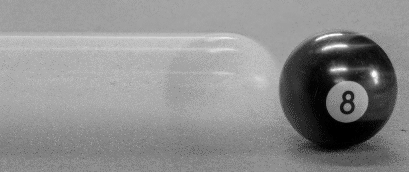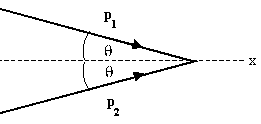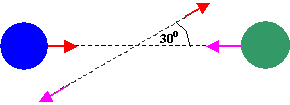## Conservation of momentum

Consider two interacting objects.  If object 1 pushes on object 2 with a force F = 10 N for 2 s to the right, then the momentum of object 2 changes by 20 Ns = 20 kg m/s to the right.  By Newton's third law object 2 pushes on object 1 with a force F = 10 N for 2 s to the left.  The momentum of object 1 changes by 20 Ns = 20 kg m/s to the left.  The total momentum of both objects does not change.  For this reason we say that the total momentum of the interacting objects is conserved.

Newton's third law implies that the total momentum of a system of interacting objects not acted on by outside forces is conserved.

The total momentum in the universe is conserved.  The momentum of a single object, however, changes when a net force acts on the object for a finite time interval.  Conversely, if no net force acts on an object, its momentum is constant.

For a system of objects, a component of the momentum along a chosen direction is constant, if no net outside force with a component in this chosen direction acts on the system.

### CollisionsIn collisions between two isolated objects Newton's third law implies that momentum is always conserved.  In collisions, it is assumed that the colliding objects interact for such a short time, that the impulse due to external forces is negligible.  Thus the total momentum of the system just before the collision is the same as the total momentum just after the collision.
Collisions in which the kinetic energy is also conserved, i.e. in which the kinetic energy just after the collision equals the kinetic energy just before the collision, are called elastic collision.  In these collisions no ordered energy is converted into thermal energy.
Collisions in which the kinetic energy is not conserved, i.e. in which some ordered energy is converted into internal energy, are called inelastic collisions.  If the two objects stick together after the collision and move with a common velocity vf, then the collision is said to be perfectly inelastic.

Note:
In collisions between two isolated objects momentum is always conserved.
Kinetic energy is only conserved in elastic collisions.

We always have m1v1i + m2v2i = m1v1f + m2v2f.
Only for elastic collisions do we also have ½m1v1i2 + ½m2v2i2 = ½m1v1f2 + ½m2v2f2.

#### Problem:

If two objects collide and one is initially at rest, is it possible for both to be at rest after the collision?  Is it possible for one to be at rest after the collision?  Explain!

Solution:

• Reasoning:
In collisions between two objects momentum is conserved.  Since the initial momentum is not zero, the final momentum is not zero.  Both objects cannot be at rest.
It is possible for one of the objects to be at rest after the collision.  For example, if the masses of the two objects are equal, then after a head-on elastic collision the object initially at rest is moving and the object initially moving is at rest.

#### Problem:

A 10 g bullet is stopped in a block of wood (m = 5 kg).  The speed of the bullet-wood combination immediately after the collision is 0.6 m/s.  What was the original speed of the bullet?

Solution:

• Reasoning:
This is a perfectly inelastic collision.  The two objects stick together after the collision and move with a common velocity vf.  Assume the motion is along the x-direction.  We then have
m1v1 + m2v2 = (m1 + m2)vf.
m1 is the mass of the bullet, v1 its initial velocity, m2 is the mass of the block, v2 its initial velocity.
• Details of the calculation:
Initially the block is at rest, v2 = 0.  Therefore
(0.01 kg)v1 = (5.01 kg)(0.6 m/s).  v1 = 300.6 m/s.

#### Problem:

Two cars of equal mass and equal speeds collide head on.  Do they experience a greater force if the collision is elastic or perfectly inelastic and they stick together?

Solution:

• Reasoning:
Let the cars move along the z-axis.  When the collision is elastic, the change in momentum of the car moving initially in the positive x-direction is pfinal - pinitial = -2 pinitial, since the car bounces back and  pfinal = -pinitial.  When the collision is inelastic, the cars come to rest and the change in momentum of the car moving initially in the positive x-direction is pfinal - pinitial = pinitial, since pfinal = 0.  So the impulse has twice the magnitude in the elastic collision.  If the collisions last about the same amount of time, then the average force experienced by the car is twice as large in the elastic collision.

#### Problem:

A 90 kg fullback running east with a speed of 5 m/s is tackled by a 95 kg opponent running north with a speed of 3 m/s.  If the collision is perfectly inelastic, calculate the speed and the direction of the players just after the tackle.

Solution:

• Reasoning:
Momentum is a vector.  In the collision, the total momentum is conserved.
• Details of the calculation:
The initial momentum of player 1 is p1 = (90 kg)(5 m/)s i = 450 kgm/s i.
The initial momentum of player 2 is p2 = (95 kg)(3m/s) j = 285 kgm/s j.
Momentum is conserved, the final momentum p of both players is p = p1 + p2.
p = (m1 + m2)v.
v = 2.432 m/s i + 1.54 m/s j.
v2 = 8.29 (m/s)2, v = 2.88 m/s.
The speed of the players after the collision is 2.88 m/s.
tanθ = py/px = 285/450 = 0.63, θ = 32.34o.
Their direction of travel makes an angle θ = 32.34o with the x-axis.  (The x-axis is pointing east.)

#### Problem:

A 30,000 kg freight car is coasting at 0.850 m/s with negligible friction under a hopper that dumps 110,000 kg of scrap metal into it.  What is the final velocity of the loaded freight car?

Solution:

• Reasoning:
For a system of objects, a component of the momentum along a chosen direction is constant, if no net outside force with a component in this chosen direction acts on the system.
• Details of the calculation:
Let the direction of motion of the car be the positive x-direction.
Before the dump the momentum of the car was in the x-direction with magnitude p = mv = 2.55*104 kg m/s.
The x- component of the momentum of the scrap metal was zero,
so the total initial momentum in the x-direction was pi = 2.55*104 kgm/s.
No external force acts on the objects in the x-direction, therefore the x-component of the total momentum is conserved.
pf = (mcar + mmetal)vf = (140,000 kg)vf = pi = 2.55*104 kgm/s.
vf = (2.55*104 kgm/s)/(140,000 kg)= 0.182 m/s.

#### Problem:

After a completely inelastic collision between two objects of equal mass, each having initial speed v, the two move off together with speed v/3.  What was the angle between their initial directions?

Solution:

• Reasoning:
The external force acting on the system is zero, the total momentum is conserved.
Assume that the final velocity of the objects points in the +x-direction.  Before the collision the velocity vector of both objects makes an angle θ with the x-axis as shown.• Details of the calculation:
p1x + p2x = pfx = pf,  p1y + p2y = 0.
2mv cosθ = 2mv/3, cosθ = 1/3, θ = 70.5o.
The angle between their initial directions is 2θ = 141o.

#### Problem:

The mass of the blue puck is 20% greater than the mass of the green one.  Before colliding, the pucks approach each other with equal and opposite momenta, and the green puck has an initial speed of 10 m/s.  Find the speed of the pucks after the collision, if half the kinetic energy is lost during the collision.Solution:

• Reasoning:
The collision is inelastic, since energy is not conserved.
The total momentum of the two pucks is zero before the collision and after the collision.
Let particle 1 be the green puck and particle 2 be the blue puck.  Before and after the collision the ratio of the speeds is v2/v1 = m1/m2 = 1/1.2.
The final kinetic energy of the system equals ½ times its initial kinetic energy.
½m1v1i2 + ½m2v2i2 = 2*(½m1v1f2 + ½m2v2f2).
• Details of the calculation:
½m1v1i2 + ½m2v2i2 = 2*(½m1v1f2 + ½m2v2f2).
Algebra:
m1v1i2 + m2v2i2 = 2 (m1v1f2 + m2v2f2).
m1v1i2 + 1.2 m1(v1i/1.2)2 = 2 (m1v1f2 + 1.2 m1(v1f/1.2)2).
m1(v1i2 + v1i2/1.2) = 2 m1(v1f2 + v1f2/1.2).
v1i2 = 2 v1f2, v1f = 0.707 v1i.
v1f = 7.07 m/s,  v2f = 5.89 m/s.

#### Embedded Question 1

During a visit to the International Space Station, an astronaut was positioned motionless in the center of the station, out of reach of any solid object on which he could exert a force.  Suggest a method by which he could move himself away from this position, and explain the physics involved.

Discuss this with your fellow students in the discussion forum!

#### Embedded Question 2

Football coaches advise players to block, hit, and tackle with their feet on the ground rather than by leaping through the air.  Using the concepts of momentum, work, and energy, explain how a football player can be more effective with his feet on the ground.

Discuss this with your fellow students in the discussion forum!